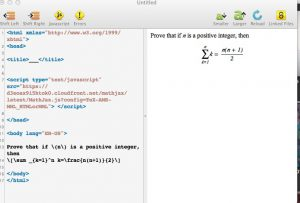# TeX in this blog

When we first got this blog, it looked as though entering mathematical notation was going to be difficult. It isn’t! MathJax to the rescue. To get the following mathematical notation, you basically need to add a script, and write your math in TeX.

Prove that if $$n$$ is a positive integer, then
$\sum _{k=1}^n k=\frac{n(n+1)}{2}$

First of all, you edit the entry with format set to “None.” Then you add the script that is shown in the screen shot, which you can copy from mathjax siteNow we still need to find some content for the blog!